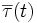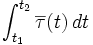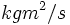# Angular impulse

## Definition

The angular impulse created by a (possibly time-varying) torque over a time period is dfefined as the integral of the torque over the time period. In other words, the angular impulse created by a torque$\overline{\tau}(t)$ from time$t = t_1$ to time$t = t_2$ is defined as the integral:$\int_{t_1}^{t_2} \overline{\tau}(t) \, dt$

## Units and dimensions

MLT dimensions$ML^2T^{-1}$: MLT;1;2;-1
SI units$J-s$ (Joule-second) or$kgm^2/s$ (kilograms peter squared per second)